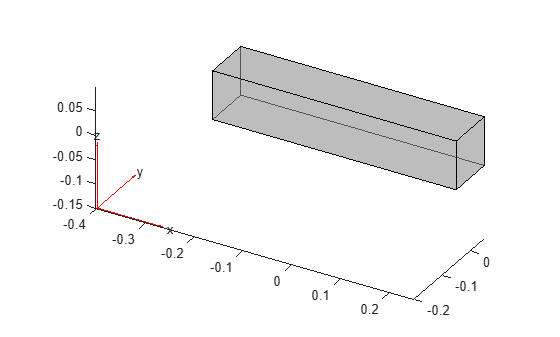Find body load assigned to geometric region

## Syntax

``bl = findBodyLoad(structuralmodel.BodyLoads,RegionType,RegionID)``

## Description

example

````bl = findBodyLoad(structuralmodel.BodyLoads,RegionType,RegionID)` returns the body load assigned to a geometric region of the structural model. A body load must use units consistent with the geometry and other model attributes.```

## Examples

collapse all

Create a structural model.

`structuralModel = createpde("structural","static-solid");`

Create and plot the geometry.

```gm = multicuboid(0.5,0.1,0.1); structuralModel.Geometry = gm; pdegplot(structuralModel,"FaceAlpha",0.5)```Specify Young's modulus, Poisson's ratio, and the mass density. Notice that the mass density value is required for modeling gravitational effects.

```structuralProperties(structuralModel,"YoungsModulus",210E3, ... "PoissonsRatio",0.3,... "MassDensity",2.7E-6);```

Specify the gravity load on the beam.

```structuralBodyLoad(structuralModel, ... "GravitationalAcceleration",[0;0;-9.8]);```

Check the body load specification for cell 1.

`findBodyLoad(structuralModel.BodyLoads,"Cell",1)`
```ans = BodyLoadAssignment with properties: RegionType: 'Cell' RegionID: 1 GravitationalAcceleration: [3x1 double] AngularVelocity: [] Temperature: [] TimeStep: [] Label: [] ```

## Input Arguments

collapse all

Body loads of the model, specified as a `BodyLoads` property of a `StructuralModel` object.

Geometric region type, specified as `"Face"` for a 2-D model or `"Cell"` for a 3-D model.

Example: `findBodyLoad(structuralmodel.BodyLoads,"Cell",1)`

Data Types: `char` | `string`

Geometric region ID, specified as a vector of positive integers. Find the region IDs by using `pdegplot`.

Example: `findBodyLoad(structuralmodel.BodyLoads,"Cell",1)`

Data Types: `double`

## Output Arguments

collapse all

Body load assignment, returned as a `BodyLoadAssignment` object. For details, see BodyLoadAssignment Properties.

## Version History

Introduced in R2017b